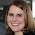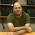## Sunday, October 29, 2017

### Statistics Sunday: Likelihood versus Probability

I recently finished reading Inference and Disputed Authorship: The Federalist by Frederick Mosteller and David L. Wallace. This book, which details the study Mosteller and Wallace did to determine (statistically) who authored the disputed Federalist papers, was highly recommended as a good primer on not only authorship studies but Bayesian inference.

As is often the case, this book frequently used a term I see in many statistical texts: likelihood. I breezed over this word multiple times (as I usually do) before I finally stopped and really considered it. (Which I do now, usually before saying, "Ooo, would this make a good blog post?")

Likelihood is a term used often in statistics, but I realized I wasn't completely clear on this concept, or rather, how it differed from related concepts, like odds or probability. If it meant the same thing as, say, probability, why use this other term? And why use it in seemingly specific ways?

It turns out that these terms are, in fact, different from each other, but they reflect related concepts. As with many statistics concepts (like degrees of freedom - see posts here and here), there are simple ways to describe these concepts, and more difficult ways.

First, the simple way. Probability deals with the chance that something will happen. That is, it is generated beforehand. When I flip a coin, I know the probability of heads is 50%. And I can use what I know about probability to determine chance of a certain string of events. Likelihood deals with something that already happened, and gets at the inference for the thing that happened. So if I flip a coin 20 times and get heads each time, you might want to discuss the likelihood that I'm using a fair coin. (Maybe I'm using a double-headed coin, for instance.)

Now, the more complex way. Likelihood is specifically related to our use of data to derive underlying truths. Remember that when we conduct statistical analyses, we're often using sample data to estimate underlying population values (parameters). We may never know what those parameters actually are, because we can't measure everyone in the population, or because of measurement error, or any number of explanations. We can only estimate those values.

We know that sample data can be biased in a number of ways, and we have different corrections to help us turn those sample values (statistics) into estimated population values (parameters). We want to make sure that we estimate population values that make sense with our sample data. We're never going to get sample data that exactly matches population values, so there will be margins of error, but we want our sample data to have a high chance of occurring given our estimated population value is correct. This concept - the chance of observing our sample data given the estimated population value is correct - is likelihood.

In the coming weeks, check back for a post discussing an application of this concept of likelihood: maximum likelihood estimation!

1.Finally someone blogging about likelihood approach to statistics :)

1.Thanks for stopping by! This is a concept I've been meaning to blog about, but I struggle with taking my understanding of it and translating it to plain language descriptions. So this blog is in part a chance to teach and in another part, a chance to improve my own understanding. I blog about statistics twice a week - hope you'll stop by again!

2.So, to check my understanding of what you are saying...

Probability is the p value that I set ahead of time.
Is likelihood, then, the obtained p value from my analyses?

Also, likelihood is the margin of error, such as the mean is 12.4 plus or minus 3.7 for 90% likelihood that the population mean is within this range? Yes?

1.Not exactly, but margins of error do come into play. So likelihood is in reference to statistics (the values you obtain from your sample) and parameters (the population value you're estimating). P-values are used to describe and test your statistics, but they aren't really statistics themselves. For example, if I did a public opinion poll on approval rating of the President, I'll obtain values from my sample (proportion approving, etc.) that I use to estimate the true population value (proportion of Americans approving). Likelihood refers to the chance that my estimated value represents the true underlying population value.

That's a pretty straightforward example, but likelihood can be used in more complex situations, such as iterative analysis techniques that start with an estimated value then compare back to the data to derive better and better estimates. I'll talk more about this technique soon!

3.On a related question...it is hard enough getting students to understand confidence intervals. How do you explain why we should use 90% or 95% CI?

4.The likelihood function in statistics exchanges the role of the parameters and random variable. In a probability situation the parameters are assumed to be fixed (though they may be unknown), from which one can determine probabilities of the random variable. In the theory of likelihood as formed by R. A. Fisher, one holds the data fixed, chooses a model (this is key), and then studies the likelihood function. For instance, maximum likelihood estimation looks at the maximum of the likelihood function to find the value of the parameter that maximizes it. Likelihoods are essentially ratios of probabilities and do not sum/integrate to 1. The likelihood also plays a role in Bayesian statistics because posterior is proportional to likelihood*prior.

In reality and in most problems, we use the log-likelihood rather than the likelihood. Deep results in mathematics and mathematical statistics suggest that as sample sizes grow, the log-likelihood behaves like a quadratic, which relates to the central limit theorem and justifies the Wald interval.

I highly recommend the book In All Likelihood by Yudi Pawitan, which is readable and has numerous examples, though it does require some knowledge of calculus and mathematical statistics.

1.